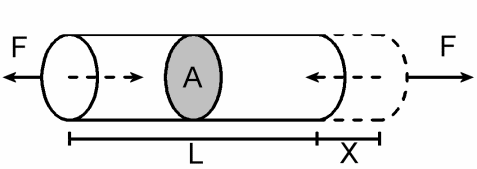## Symbols and units

The table below identifies the symbols and units used in the calculation of stress and strain.

 Description Symbol Name Units Direct stress σ Sigma N/m² and N/mm² Direct strain ε Epsilon None Shear stress τ Tau N/m² and N/mm² Young’s modulus of elasticity E N/m² and N/mm²

Note: 1N/mm²  =  10⁶N/m² = 1MN/m²
And 1kN/mm² = 1GN/m²
The alternative for stress is the pascal (pa)which equals 1 N/m²

Two effects may be identified, when the force acts on a solid material which remains stationary.

The material will:-

• Exert an internal resisting force known as a state of stress;
• Experience dimensional changes

This behaviour is typical of stressed engineering components but the dimensional changes are usually small are not normally seen with the naked eye.

## Direct forces

One end of a bar may be subjected to push or pull.  Now, if a bar remains stationary, a pull on one end must result in an equal and opposite pull on the other end, and the bar is said to be in tension.  Similarly, a push on one end is accompanied by a push on the other end, and the bar is in compression.

The forces which are producing a tension or compression are called direct forces.

Also, direct forces are called either tensile (A pull) or compressive (A push).

Tensile forces cause a bar to stretch and compressive forces cause a bar to contract.

Fig 1Fig 1 Illustrates a bar acted upon by a tensile force at either end causing the bar to stretch.

F = The applied force
A = Cross sectional area of the bar
L = Original length of the bar
x = Change in length produced by the applied force, F

## Stress

Forces on a component, e.g.  A bar, are often referred to as loads.
The load that a particular component can withstand will depend on the dimensions of the component as well as the material it is made from; in particular its cross sectional area when subjected to direct forces.

For example if the cross sectional area is doubled then the load that the component can withstand will be doubled.
Therefore what is important is the intensity of loading i.e.  The load per unit area, and this is given the name direct stress.  Often the word ‘direct’ is omitted.

Hence

Stress = Force
Area

Or in symbols

σ = F
A

Example 1: A rectangular bar of dimensions 5mm breadth x 20 mm depth, supports a load of 4900 N. Determine the stress in the bar.

Known values

F = 4900 N and A = 5 x 20 = 100mm²

Then σ = 4900 = 49 N/mm²
100

## Strain

In addition to controlling the stress produced in a component, the dimensional changes also require consideration.
In the case of a bar loaded in tension the extension of the bar depends upon its total length. The bar is said to be strained and the strain is defined as the fractional change in the length of the bar.

Hence

Strain = Change in length
Original length

Note strain does not have any units.

Example 2: A wire of length 2 m, extends by 0.25 mm when acted upon by a tensile force. Determine the strain in the wire.

From ε = x
l

ε = 0.25
2 x 10³

= 1.25 x 10¯⁴

Explore posts in the same categories: VIBRATION